# Double-periodic function

A single-valued analytic functionwith only isolated singularities on the entire finite complex-plane, and such that there exists two numbers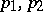whose quotient is not a real number and which are periods of, i.e.are such that the identity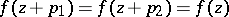is valid. (Ifis real and rational,is a simply-periodic function; ifis real and irrational,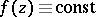.) All numbers of the form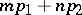where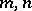are integers are also periods of. All periods of a given double-periodic function form a discrete Abelian group with respect to addition, known as the period group (or the period module), a basis of which (a period basis) is constituted by two primitive periods,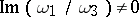. All remaining periods of this double-periodic function may be represented in the formwhereare integers. Analytic functions of one complex variable with more than two primitive periods do not exist, except for constants.

The points of the formwhereare integers form the period lattice (subdividing the entire-plane into period parallelograms). Points (numbers)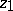,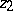for which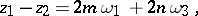are said to be congruent (comparable with respect to the period module). At congruent points the double-periodic functionassumes the same value, so that it is sufficient to study the behaviour ofin some basic period parallelogram. This is usually the set of pointsi.e. the parallelogram with verticesA non-constant double-periodic function that is regular in the entire basic period parallelogram does not exist. Meromorphic double-periodic functions are called elliptic functions (cf. Elliptic function). The generalization of the concept of an elliptic function to include functions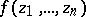of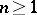complex variables are called Abelian functions (cf. Abelian function).